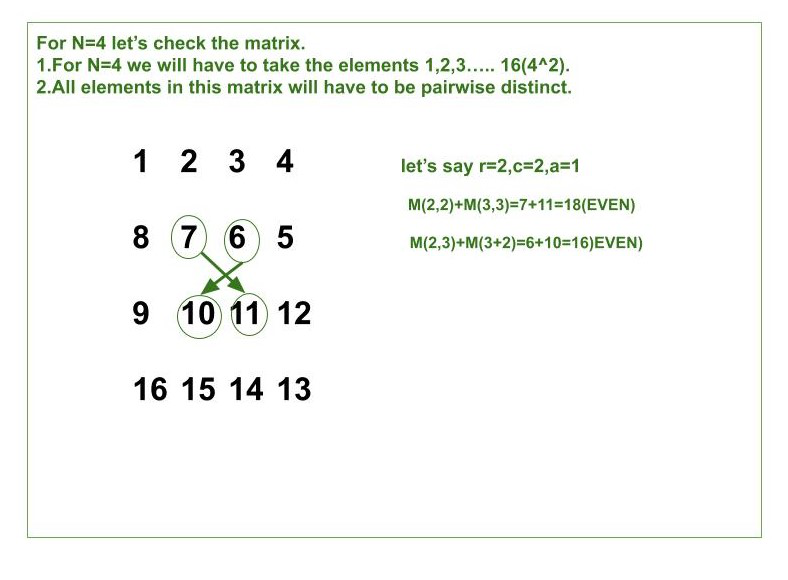# Construct a Matrix N x N with first N^2 natural numbers for an input N

• Difficulty Level : Medium
• Last Updated : 26 Apr, 2021

Given an integer N, the task is to construct a matrix M[][] of size N x N with numbers in the range [1, N^2] with the following conditions :

1. The elements of the matrix M should be an integer between 1 and N^2.
2. All elements of the matrix M are pairwise distinct.
3. For each square submatrix containing cells in row r through r+a and in columns c through c+a(inclusive) for some valid integers r,c and a>=0: M(r,c)+M(r+a,c+a) is even and M(r,c+a)+M(r+a,c) is even.

Examples:

Input: N = 2
Output:
1 2
4 3
Explanation:
This matrix has 5 square submatrix and 4 of them (, , , ) have a=0 so they satisfy the conditions.
The last square submatrix is the whole matrix M where r=c=a=1. We can see that M(1, 1)+M(2, 2)=1+3=4 and M(1, 2)+M(2, 1)=2+4=6 are both even.
Input: N = 4
Output:
1 2 3 4
8 7 6 5
9 10 11 12
16 15 14 13

Approach: We know that the sum of two numbers is even when their parity is the same. Let us say the parity of M(i, j) is odd that means the parity of M(i+1, j+1), M(i+1, j-1), M(i-1, j+1), M(i-1, j-1) has to be odd.
Below is the illustration for N = 4 to generate a matrix of size 4×4:So from the above illustration we have to fill the matrix in the Checkerboard Pattern. We can fill it in two ways:

• All black cells have an odd integer and white cells have an even integer.
• All black cells have an even integer and white cells have an odd integer.

Below is the implementation of the above approach:

## C++

 `// C++ program for the above approach``#include``using` `namespace` `std;` `// Function to print the desired matrix``void` `UniqueMatrix(``int` `N)``{``    ``int` `element_value = 1;``    ``int` `i = 0;` `    ``// element_value will start from 1``    ``// and go up to N ^ 2` `    ``// i is row number and it starts``    ``// from 0 and go up to N-1``    ` `    ``// Iterate ove all [0, N]``    ``while``(i < N)``    ``{``        ` `        ``// If is even``        ``if``(i % 2 == 0)``        ``{``            ``for``(``int` `f = element_value;``                    ``f < element_value + N; f++)``            ``{``                ` `                ``// If row number is even print``                ``// the row in forward order``                ``cout << f << ``" "``;``            ``}``            ``element_value += N;``        ``}``        ``else``        ``{``            ``for``(``int` `k = element_value + N - 1;``                    ``k > element_value - 1; k--)``            ``{``                ` `                ``// If row number is odd print``                ``// the row in reversed order``                ``cout << k << ``" "``;` `            ``}``            ``element_value += N;``        ``}``        ``cout << endl;``        ``i = i + 1;``    ``}``}` `// Driver Code``int` `main()``{``    ` `    ``// Given matrix size``    ``int` `N = 4;` `    ``// Function call``    ``UniqueMatrix(N);``}` `// This code is contributed by chitranayal`

## Java

 `// Java program for the above approach``public` `class` `Gfg``{``  ` `    ``// Function to print the desired matrix``    ``public` `static` `void` `UniqueMatrix(``int` `N)``    ``{``        ``int` `element_value = ``1``;``        ``int` `i = ``0``;``        ` `        ``// element_value will start from 1``        ``// and go up to N ^ 2``  ` `        ``// i is row number and it starts``        ``// from 0 and go up to N-1``      ` `        ``// Iterate ove all [0, N]``        ``while``(i < N)``        ``{``            ``// If is even``            ``if``(i % ``2` `== ``0``)``            ``{``                ``for``(``int` `f = element_value;``                    ``f < element_value + N; f++)``                ``{``                  ` `                    ``// If row number is even print``                    ``// the row in forward order``                    ``System.out.print(f+``" "``);``                ``}``                ``element_value += N;``            ``}``            ``else``            ``{``                ``for``(``int` `k = element_value + N - ``1``;``                    ``k > element_value - ``1``; k--)``                ``{``                  ` `                    ``// If row number is odd print``                    ``// the row in reversed order``                    ``System.out.print(k+``" "``);``                ``}``                ``element_value += N;``            ``}``            ``System.out.println();``            ``i = i + ``1``;``        ``}``    ``}``  ` `    ``// Driver Code``    ``public` `static` `void` `main(String []args)``    ``{``      ` `        ``// Given matrix size``        ``int` `N = ``4``;``      ` `        ``// Function call``        ``UniqueMatrix(N);``    ``}``}` `// This code is contributed by avanitrachhadiya2155`

## Python3

 `# Python3 program for the above approach` `# Function to print the desired matrix``def` `UniqueMatrix(N):` `    ``element_value ``=` `1``    ``i ``=` `0` `    ``# element_value will start from 1``    ``# and go up to N ^ 2` `    ``# i is row number and it starts``    ``# from 0 and go up to N-1``    ` `    ``# Iterate ove all [0, N]``    ``while``(i < N):``        ` `        ``# If is even``        ``if``(i ``%` `2` `=``=` `0``):` `            ``for` `f ``in` `range``(element_value, element_value ``+` `N, ``1``):` `                ``# If row number is even print``                ``# the row in forward order``                ``print``(f, end ``=``' '``)``            ``element_value ``+``=` `N` `        ``else``:` `            ``for` `k ``in` `range``(element_value ``+` `N``-``1``, element_value``-``1``, ``-``1``):` `                ``# if row number is odd print``                ``# the row in reversed order``                ``print``(k, end ``=``' '``)` `            ``element_value ``+``=` `N` `        ``print``()``        ``i ``=` `i ``+` `1`  `# Driver Code` `# Given Matrix Size``N ``=` `4` `# Function Call``UniqueMatrix(N)`

## C#

 `// C# program for the above approach``using` `System;``class` `GFG``{``    ``static` `void` `UniqueMatrix(``int` `N)``    ``{``        ``int` `element_value = 1;``        ``int` `i = 0;``         ` `        ``// element_value will start from 1``        ``// and go up to N ^ 2``   ` `        ``// i is row number and it starts``        ``// from 0 and go up to N-1``       ` `        ``// Iterate ove all [0, N]``        ``while``(i < N)``        ``{``          ` `            ``// If is even``            ``if``(i % 2 == 0)``            ``{``                ``for``(``int` `f = element_value;``                    ``f < element_value + N; f++)``                ``{``                  ` `                    ``// If row number is even print``                    ``// the row in forward order``                    ``Console.Write(f + ``" "``);``                ``}``                ``element_value += N;``            ``}``            ``else``            ``{``                ``for``(``int` `k = element_value + N - 1;``                    ``k > element_value - 1; k--)``                ``{``                    ``Console.Write(k + ``" "``);``                ``}``                ``element_value += N;``            ``}``            ``Console.WriteLine();``            ``i = i + 1;``        ``}``    ``}``  ` `  ``// Driver code``  ``static` `public` `void` `Main ()``  ``{``    ``// Given matrix size``    ``int` `N = 4;` `    ``// Function call``    ``UniqueMatrix(N);``  ``}``}` `// This code is contributed by rag2127`

## Javascript

 ``
Output:
```1 2 3 4
8 7 6 5
9 10 11 12
16 15 14 13```

Time Complexity: O(N^2)
Auxiliary Space: O(N^2)

My Personal Notes arrow_drop_up